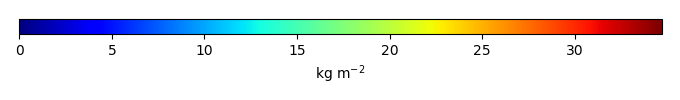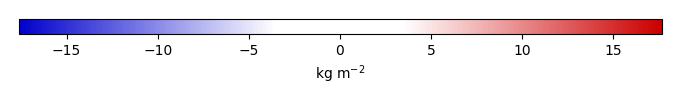# Mean State

Period Mean (original grids) [Pg]
Model Period Mean (intersection) [Pg]
Model Period Mean (complement) [Pg]
Benchmark Period Mean (intersection) [Pg]
Benchmark Period Mean (complement) [Pg]
Bias [kg m-2]
Bias Score 
Spatial Distribution Score 
Overall Score 
Benchmark [-] 64.0
CLM4 [-] 49.3 50.0 0.362 63.9 0.0663 -3.82 0.715 0.791 0.753
CLM4.5 [-] 45.2 45.1 0.424 63.9 0.0663 -5.16 0.703 0.846 0.775
CLM5 [-] 38.4 38.4 0.429 63.9 0.0663 -7.15 0.634 0.580 0.607
Period Mean (original grids) [Pg]
Model Period Mean (intersection) [Pg]
Model Period Mean (complement) [Pg]
Benchmark Period Mean (intersection) [Pg]
Benchmark Period Mean (complement) [Pg]
Bias [kg m-2]
Bias Score 
Spatial Distribution Score 
Overall Score 
Benchmark [-] 3.34
CLM4 [-] 7.61 5.85 0.958 3.34 0.00700 0.882 0.535 0.838 0.687
CLM4.5 [-] 2.24 1.33 0.706 3.34 0.00700 -0.628 0.471 0.372 0.422
CLM5 [-] 3.30 2.21 0.738 3.34 0.00700 -0.331 0.497 0.570 0.533
Period Mean (original grids) [Pg]
Model Period Mean (intersection) [Pg]
Model Period Mean (complement) [Pg]
Benchmark Period Mean (intersection) [Pg]
Benchmark Period Mean (complement) [Pg]
Bias [kg m-2]
Bias Score 
Spatial Distribution Score 
Overall Score 
Benchmark [-] 14.1
CLM4 [-] 10.9 9.99 0.716 14.1 0.0194 -0.481 0.543 0.745 0.644
CLM4.5 [-] 5.23 4.36 0.756 14.1 0.0194 -1.29 0.464 0.536 0.500
CLM5 [-] 12.3 11.1 0.996 14.1 0.0194 -0.223 0.547 0.791 0.669
Period Mean (original grids) [Pg]
Model Period Mean (intersection) [Pg]
Model Period Mean (complement) [Pg]
Benchmark Period Mean (intersection) [Pg]
Benchmark Period Mean (complement) [Pg]
Bias [kg m-2]
Bias Score 
Spatial Distribution Score 
Overall Score 
Benchmark [-] 1.22
CLM4 [-] 0.802 0.743 0.0853 1.22 0.00453 -0.142 0.548 0.753 0.650
CLM4.5 [-] 3.11 2.74 0.386 1.22 0.00453 0.689 0.482 0.744 0.613
CLM5 [-] 4.18 3.37 0.817 1.22 0.00453 0.991 0.469 0.503 0.486
Period Mean (original grids) [Pg]
Model Period Mean (intersection) [Pg]
Model Period Mean (complement) [Pg]
Benchmark Period Mean (intersection) [Pg]
Benchmark Period Mean (complement) [Pg]
Bias [kg m-2]
Bias Score 
Spatial Distribution Score 
Overall Score 
Benchmark [-] 47.3
CLM4 [-] 63.3 60.7 2.35 45.9 1.37 10.2 0.677 0.919 0.798
CLM4.5 [-] 54.1 51.7 2.13 45.9 1.37 7.51 0.711 0.793 0.752
CLM5 [-] 40.1 38.1 1.81 45.9 1.37 2.33 0.683 0.514 0.598
Period Mean (original grids) [Pg]
Model Period Mean (intersection) [Pg]
Model Period Mean (complement) [Pg]
Benchmark Period Mean (intersection) [Pg]
Benchmark Period Mean (complement) [Pg]
Bias [kg m-2]
Bias Score 
Spatial Distribution Score 
Overall Score 
Benchmark [-] 41.6
CLM4 [-] 26.7 25.9 0.164 41.6 0.0303 -1.67 0.588 0.821 0.704
CLM4.5 [-] 31.5 30.7 0.274 41.6 0.0303 -1.10 0.646 0.903 0.774
CLM5 [-] 52.3 51.1 0.450 41.6 0.0303 1.23 0.552 0.742 0.647
Period Mean (original grids) [Pg]
Model Period Mean (intersection) [Pg]
Model Period Mean (complement) [Pg]
Benchmark Period Mean (intersection) [Pg]
Benchmark Period Mean (complement) [Pg]
Bias [kg m-2]
Bias Score 
Spatial Distribution Score 
Overall Score 
Benchmark [-] 15.6
CLM4 [-] 40.7 38.6 2.67 15.6 0.0349 2.91 0.449 0.508 0.478
CLM4.5 [-] 40.8 38.2 3.05 15.6 0.0349 2.92 0.455 0.490 0.472
CLM5 [-] 31.8 29.6 2.96 15.6 0.0349 1.93 0.506 0.676 0.591
Period Mean (original grids) [Pg]
Model Period Mean (intersection) [Pg]
Model Period Mean (complement) [Pg]
Benchmark Period Mean (intersection) [Pg]
Benchmark Period Mean (complement) [Pg]
Bias [kg m-2]
Bias Score 
Spatial Distribution Score 
Overall Score 
Benchmark [-] 14.8
CLM4 [-] 28.6 26.4 1.31 14.6 0.136 2.95 0.497 0.608 0.553
CLM4.5 [-] 23.3 21.2 1.33 14.6 0.136 2.21 0.468 0.558 0.513
CLM5 [-] 24.9 22.6 1.43 14.6 0.136 2.37 0.529 0.734 0.632
Period Mean (original grids) [Pg]
Model Period Mean (intersection) [Pg]
Model Period Mean (complement) [Pg]
Benchmark Period Mean (intersection) [Pg]
Benchmark Period Mean (complement) [Pg]
Bias [kg m-2]
Bias Score 
Spatial Distribution Score 
Overall Score 
Benchmark [-] 1.78
CLM4 [-] 0.737 0.697 0.122 1.78 -0.540 0.503 0.466 0.485
CLM4.5 [-] 3.23 2.60 0.782 1.78 0.399 0.609 0.746 0.677
CLM5 [-] 6.90 5.31 1.98 1.78 1.79 0.402 0.575 0.489
Period Mean (original grids) [Pg]
Model Period Mean (intersection) [Pg]
Model Period Mean (complement) [Pg]
Benchmark Period Mean (intersection) [Pg]
Benchmark Period Mean (complement) [Pg]
Bias [kg m-2]
Bias Score 
Spatial Distribution Score 
Overall Score 
Benchmark [-] 15.3
CLM4 [-] 8.06 6.86 1.10 14.8 0.505 -0.972 0.499 0.752 0.625
CLM4.5 [-] 8.12 6.70 1.35 14.8 0.505 -0.953 0.501 0.743 0.622
CLM5 [-] 11.3 9.25 1.86 14.8 0.505 -0.174 0.552 0.810 0.681
Period Mean (original grids) [Pg]
Model Period Mean (intersection) [Pg]
Model Period Mean (complement) [Pg]
Benchmark Period Mean (intersection) [Pg]
Benchmark Period Mean (complement) [Pg]
Bias [kg m-2]
Bias Score 
Spatial Distribution Score 
Overall Score 
Benchmark [-] 13.1
CLM4 [-] 5.45 5.02 0.345 13.1 0.0236 -1.34 0.484 0.317 0.400
CLM4.5 [-] 11.3 10.4 0.655 13.1 0.0236 -0.123 0.591 0.732 0.662
CLM5 [-] 18.2 17.0 0.899 13.1 0.0236 1.50 0.498 0.694 0.596
Period Mean (original grids) [Pg]
Model Period Mean (intersection) [Pg]
Model Period Mean (complement) [Pg]
Benchmark Period Mean (intersection) [Pg]
Benchmark Period Mean (complement) [Pg]
Bias [kg m-2]
Bias Score 
Spatial Distribution Score 
Overall Score 
Benchmark [-] 455.
CLM4 [-] 467. 453. 14.8 449. 5.38 0.561 0.628 0.888 0.758
CLM4.5 [-] 453. 434. 18.3 449. 5.38 0.441 0.662 0.899 0.781
CLM5 [-] 487. 464. 23.3 449. 5.38 0.776 0.637 0.859 0.748
Period Mean (original grids) [Pg]
Model Period Mean (intersection) [Pg]
Model Period Mean (complement) [Pg]
Benchmark Period Mean (intersection) [Pg]
Benchmark Period Mean (complement) [Pg]
Bias [kg m-2]
Bias Score 
Spatial Distribution Score 
Overall Score 
Benchmark [-] 0.854
CLM4 [-] 1.25 0.783 0.466 0.849 0.00531 0.0569 0.510 0.681 0.595
CLM4.5 [-] 1.28 0.708 0.583 0.849 0.00531 0.0261 0.504 0.508 0.506
CLM5 [-] 1.51 0.664 0.856 0.849 0.00531 0.0462 0.484 0.722 0.603
Period Mean (original grids) [Pg]
Model Period Mean (intersection) [Pg]
Model Period Mean (complement) [Pg]
Benchmark Period Mean (intersection) [Pg]
Benchmark Period Mean (complement) [Pg]
Bias [kg m-2]
Bias Score 
Spatial Distribution Score 
Overall Score 
Benchmark [-] 2.82
CLM4 [-] 3.07 2.65 0.393 2.82 0.00299 -0.0258 0.565 0.698 0.631
CLM4.5 [-] 7.91 6.65 1.14 2.82 0.00299 1.24 0.498 0.816 0.657
CLM5 [-] 14.5 12.3 2.08 2.82 0.00299 3.01 0.268 0.346 0.307
Period Mean (original grids) [Pg]
Model Period Mean (intersection) [Pg]
Model Period Mean (complement) [Pg]
Benchmark Period Mean (intersection) [Pg]
Benchmark Period Mean (complement) [Pg]
Bias [kg m-2]
Bias Score 
Spatial Distribution Score 
Overall Score 
Benchmark [-] 4.95
CLM4 [-] 4.63 3.96 0.391 4.88 0.0615 0.376 0.455 0.454 0.454
CLM4.5 [-] 8.50 7.65 0.586 4.88 0.0615 2.21 0.428 0.417 0.422
CLM5 [-] 10.2 9.16 0.789 4.88 0.0615 3.11 0.449 0.515 0.482
Period Mean (original grids) [Pg]
Model Period Mean (intersection) [Pg]
Model Period Mean (complement) [Pg]
Benchmark Period Mean (intersection) [Pg]
Benchmark Period Mean (complement) [Pg]
Bias [kg m-2]
Bias Score 
Spatial Distribution Score 
Overall Score 
Benchmark [-] 13.0
CLM4 [-] 13.1 12.6 0.328 13.0 0.00854 0.140 0.653 0.847 0.750
CLM4.5 [-] 14.3 13.8 0.399 13.0 0.00854 0.479 0.639 0.899 0.769
CLM5 [-] 11.8 11.4 0.332 13.0 0.00854 -0.100 0.652 0.874 0.763
Period Mean (original grids) [Pg]
Model Period Mean (intersection) [Pg]
Model Period Mean (complement) [Pg]
Benchmark Period Mean (intersection) [Pg]
Benchmark Period Mean (complement) [Pg]
Bias [kg m-2]
Bias Score 
Spatial Distribution Score 
Overall Score 
Benchmark [-] 21.4
CLM4 [-] 16.5 16.2 0.296 21.3 0.128 -0.772 0.622 0.789 0.706
CLM4.5 [-] 28.3 28.0 0.400 21.3 0.128 1.61 0.613 0.900 0.757
CLM5 [-] 43.8 43.5 0.471 21.3 0.128 4.72 0.474 0.788 0.631
Period Mean (original grids) [Pg]
Model Period Mean (intersection) [Pg]
Model Period Mean (complement) [Pg]
Benchmark Period Mean (intersection) [Pg]
Benchmark Period Mean (complement) [Pg]
Bias [kg m-2]
Bias Score 
Spatial Distribution Score 
Overall Score 
Benchmark [-] 9.57
CLM4 [-] 3.96 3.74 0.266 9.53 0.0397 -1.71 0.514 0.285 0.399
CLM4.5 [-] 2.93 2.71 0.257 9.53 0.0397 -1.99 0.468 0.259 0.364
CLM5 [-] 3.09 2.90 0.233 9.53 0.0397 -1.92 0.486 0.493 0.489
Period Mean (original grids) [Pg]
Model Period Mean (intersection) [Pg]
Model Period Mean (complement) [Pg]
Benchmark Period Mean (intersection) [Pg]
Benchmark Period Mean (complement) [Pg]
Bias [kg m-2]
Bias Score 
Spatial Distribution Score 
Overall Score 
Benchmark [-] 151.
CLM4 [-] 160. 159. 0.457 151. 0.0403 1.24 0.685 0.920 0.803
CLM4.5 [-] 141. 141. 0.533 151. 0.0403 -0.828 0.773 0.952 0.863
CLM5 [-] 129. 128. 0.570 151. 0.0403 -2.41 0.765 0.777 0.771
Period Mean (original grids) [Pg]
Model Period Mean (intersection) [Pg]
Model Period Mean (complement) [Pg]
Benchmark Period Mean (intersection) [Pg]
Benchmark Period Mean (complement) [Pg]
Bias [kg m-2]
Bias Score 
Spatial Distribution Score 
Overall Score 
Benchmark [-] 10.7
CLM4 [-] 17.1 17.0 0.294 10.7 0.0170 1.10 0.453 0.706 0.579
CLM4.5 [-] 13.0 12.8 0.311 10.7 0.0170 0.468 0.485 0.741 0.613
CLM5 [-] 21.5 21.4 0.512 10.7 0.0170 1.83 0.517 0.807 0.662
Period Mean (original grids) [Pg]
Model Period Mean (intersection) [Pg]
Model Period Mean (complement) [Pg]
Benchmark Period Mean (intersection) [Pg]
Benchmark Period Mean (complement) [Pg]
Bias [kg m-2]
Bias Score 
Spatial Distribution Score 
Overall Score 
Benchmark [-] 4.41
CLM4 [-] 4.81 4.91 0.221 4.31 0.102 0.811 0.551 0.768 0.659
CLM4.5 [-] 5.02 4.94 0.251 4.31 0.102 0.986 0.538 0.764 0.651
CLM5 [-] 6.25 5.78 0.493 4.31 0.102 1.41 0.590 0.801 0.695

# Temporally integrated period mean

BENCHMARK MEANMODEL MEANBIASBIAS SCORESPATIAL TAYLOR DIAGRAMMODEL COLORS# Spatially integrated regional mean

MODEL COLORS# All Models

BenchmarkCLM4CLM4.5CLM5# Data Information

history: Mon Mar 21 09:05:59 2016: ncatted -a units,biomass,o,c,kg m-2 ./from_MQs_site/DATA/biomass/GEOCARBON/derived/biomass_0.5x0.5.nc tmp.nc